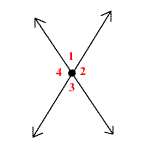# Vertical Angles

Vertical angles are a pair of opposite angles formed by intersecting lines.

In the figure, $\angle 1\text{\hspace{0.17em}}\text{\hspace{0.17em}}\text{and}\text{\hspace{0.17em}}\text{\hspace{0.17em}}\angle 3$ are vertical angles. So are $\angle 2\text{\hspace{0.17em}}\text{\hspace{0.17em}}\text{and}\text{\hspace{0.17em}}\text{\hspace{0.17em}}\angle 4$ .Vertical angles are always congruent . So, in this figure, $\angle 1\cong \angle 3$ and $\angle 2\cong \angle 4$ .